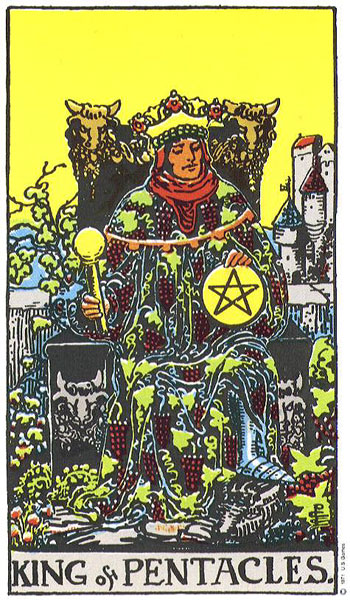## people with a 65 (King of Pentacles) life lesson

### April 5, 1970people with a 65 life lesson:Carson Palmer

born December 27th, 1979

12 + 27 +1+9+7+9 = 65 = Career.  Contracts.  Worldly success.  Making it big time.

Victoria Azarenka

born July 31st, 1989                                               7 + 31 +1+9+8+9 = 65

David Dunn

born December 27th, 1979                                 12 + 27 +1+9+7+9 = 65

Ana Konjuh

born December 27th, 1997                                 12 + 27 +1+9+9+7 = 65

Ryan Blaney

born December 31st, 1993                                  12 + 31 +1+9+9+3 = 65

Hayley Williams

born December 27th, 1988                                12 + 27 +1+9+8+8 = 65

Lauren German

born November 29th, 1978                                11 + 29 +1+9+7+8 = 65

Donna Summer

born December 31st, 1948                                  12 + 31 +1+9+4+8 = 65

John Legend

born December 28th, 1978                                 12 + 28 +1+9+7+8 = 65

Taecyeon

born December 27th, 1988                                 12 + 27 +1+9+8+8 = 65

Chamillionaire

born November 28th, 1979                                 11 + 28 +1+9+7+9 = 65

Kastro

born December 30th, 1976                                 12 + 30 +1+9+7+6 = 65

Snow

born October 30th, 1969                                      10 + 30 +1+9+6+9 = 65

Dennis Wolf

born October 30th, 1978                                      10 + 30 +1+9+7+8 = 65

Sean Paul Lockhart

born October 31st, 1986                                        10 + 31 +1+9+8+6 = 65

Ji-In Cho

born December 30th, 1976                                  12 + 30 +1+9+7+6 = 65

Ashley Graham

born October 30th, 1987                                       10 + 30 +1+9+8+7 = 65